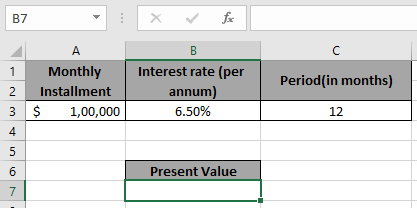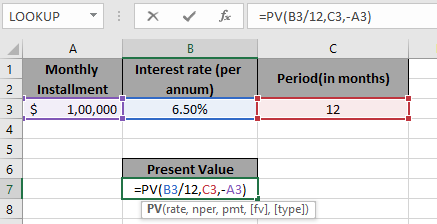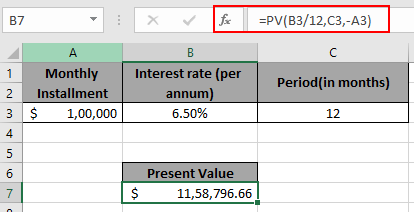# Present Value of Annuity in Excel

In this article, we will learn about how to find the Present Value of annuity using the PV function in Excel.

Present value of annuity is the present value of the fixed amount paid every month up to a period at fixed interest period
PV function returns the present value of the fixed amount paid over a period of time at a constant interest rate.

Syntax:

=PV (rate, nper, pmt, [fv], [type])

rate: Interest rate per period
nper: total no. of payment period.
pmt: amount paid each period.
[fv] - [optional] The present value of future payments must be entered as a negative number.
[type] - [optional] When payments are due. Default is 0.

Use it in example to understand it.

Here we have a data and we need to find the Present value of Annuity for the same. We have the amount of \$100,000 is paid every month over a year at a rate of 6.5%.Use the Formula:

=PV( B3/12 , C3 , -A3 )Explanation:
B3/12 : rate is divided by 12 as we are calculating interest for monthly periods.

C3 : Period, each payment made

-A3 : amount is in negative so as to get the present value in positive.

Press EnterThis means the present value of annuity of the amount paid will be \$ 11,58,796.66

Hope you understood how to find the present value of annuity of the amount using PV formula. Explore more articles here on Accounting. Please feel free to write your queries to us in the comment box below. We will help you.

Related Articles

How to Use PV function in Excel

How to Use NPV function in Excel

How to Use FV function in Excel

How to use the IPMT Function in Excel

Simple interest formula in Excel

How to calculate interest on a loan

How to use XNPV function in Excel

How to Use RATE Function in Excel

Popular Articles

50 Excel Shortcut to Increase Your Productivity

Edit a dropdown list

Absolute reference in Excel

If with conditional formatting

If with wildcards

Vlookup by date

Convert Inches To Feet and Inches in Excel 2016

Join first and last name in excel

Count cells which match either A or B

Terms and Conditions of use

The applications/code on this site are distributed as is and without warranties or liability. In no event shall the owner of the copyrights, or the authors of the applications/code be liable for any loss of profit, any problems or any damage resulting from the use or evaluation of the applications/code.